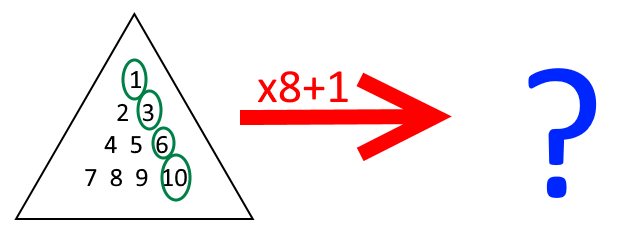### Submit a Resource

NRICH: Iff

Alison has been playing with numbers again. She started by choosing a triangular number, multiplied it by 8, and added 1. She noticed something interesting about her results…Try a few examples. Can you make a conjecture?

Once you’ve made a conjecture of your own, click below to see what Alison noticed:

“If T is a triangular number, 8T+1 is a square number.”

Can you prove the conjecture?

I wonder if there are any integers n where 8n+1 is a square number but n is not a triangular number…

Can you prove that if 8n+1 is a square number, n must be a triangular number?

Can you use your theorem to devise a quick way to check whether the following numbers are triangular numbers?

• 6214
• 3655
• 7626
• 8656

The title of this problem, “Iff”, is sometimes used by mathematicians as shorthand for “If and Only If”, which can also be represented by the double implication arrow . To explore the difference between “If”, “Only if” and “Iff”, try the problem Iffy Logic.

Age 14 to 18

Math Topics
Algebra & Pre-Algebra, Equations
High School, Educator

## Organization

NRICH (University of Cambridge)

Challenge